# 【计算机视觉】图像分割与特征提取——频域增强（低通滤波&高通滤波）> 📦个人主页：赵四司机
> 🏆学习方向：JAVA后端开发
> ⏰往期文章：SpringBoot项目整合微信支付
> 🔔博主推荐网站：牛客网 刷题|面试|找工作神器
> 📣种一棵树最好的时间是十年前，其次是现在！
> 💖喜欢的话麻烦点点关注喔，你们的支持是我的最大动力。

# 一：实验原理

``g(x,y)=h(x,y)*f(x,y)     ``

``G(u,v)=H(u,v)F(u,v)     ``

1. 计算所需增强图的傅立叶变换；
2. 将其与一个（根据需要设计的）转移函数相乘；
3. 在将结果进行傅立叶反变换以得到增强的图。

# 二：低通滤波

Butterworth低通滤波器是一种物理上可以实现的低通滤波器。n阶截断频率为d0的Butterworth低通滤波器的转移函数为：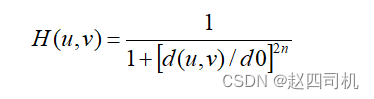``````%  实现Butterworth低通滤波器

I2=imnoise(I1,'salt');

f=double(I2);

g=fft2(f);     %采用傅立叶变换

g=fftshift(g); %数据矩阵平移

[N1,N2]=size(g);

n=2;

d0=50;

d1=5;

n1=fix(N1/2);

n2=fix(N2/2);

for i=1:N1

for j=1:N2

d=sqrt((i-n1)^2+(j-n2)^2);

%计算Butterworth低通变换函数

h=1/(1+0.414*(d/d0)^(2*n));

result(i,j)=h*g(i,j);

end

end

result=ifftshift(result);

X2=ifft2(result);

X3=uint8(real(X2));``````

# 三：高通滤波

``````% 实现Butterworth高通滤波器参考代码

%计算Butterworth高通变换函数

if d==0

h=0;

else

h=1/(1+(d1/d)^(2*n));

end

result(i,j)=h*g(i,j);

result=ifftshift(result);

X4=ifft2(result);

X5=uint8(real(X2));

figure(1),subplot(2,2,1),imshow(I1),title('原图像');

subplot(2,2,2),imshow(I2),title('加噪图像');

subplot(2,2,3),imshow(X3),

title('Butterworth低通滤波器去噪图像');

subplot(2,2,4),imshow(X5),

title('Butterworth高通滤波器去噪图像');``````

# 四：代码实战

## 2.Butterworth低通滤波器

``````function I = Butterworth_low(I_in)
f=double(I_in);
g=fft2(f);     %采用傅立叶变换
g=fftshift(g); %数据矩阵平移
[N1,N2]=size(g);
result=zeros(N1,N2);
n=2;
d0=50;
n1=fix(N1/2);
n2=fix(N2/2);
for i=1:N1
for j=1:N2
d=sqrt((i-n1)^2+(j-n2)^2);
%计算Butterworth低通变换函数
h=1/(1+0.414*(d/d0)^(2*n));
result(i,j)=h*g(i,j);
end
end
result=ifftshift(result);
X2=ifft2(result);
I=uint8(real(X2));
end

%lena图片
X1_1=Butterworth_low(I1_1);
figure,subplot(131),imshow(I1),title('lena原图像');
subplot(132),imshow(I1_1),title('加入高斯噪声');
subplot(133),imshow(X1_1),title('Butterworth低通滤波');````````````X1_2=Butterworth_low(I1_2);

figure,subplot(131),imshow(I1),title('lena原图像');

subplot(132),imshow(I1_2),title('加入椒盐噪声');

subplot(133),imshow(X1_2),title('Butterworth低通滤波');````````````X1_3=Butterworth_low(I1_3);

figure,subplot(131),imshow(I1),title('lena原图像');

subplot(132),imshow(I1_3),title('加入泊松噪声');

subplot(133),imshow(X1_3),title('Butterworth低通滤波');````````````%pout图片

X2_1=Butterworth_low(I2_1);

figure,subplot(131),imshow(I2),title('pout原图像');

subplot(132),imshow(I2_1),title('加入高斯噪声');

subplot(133),imshow(X2_1),title('Butterworth低通滤波');``````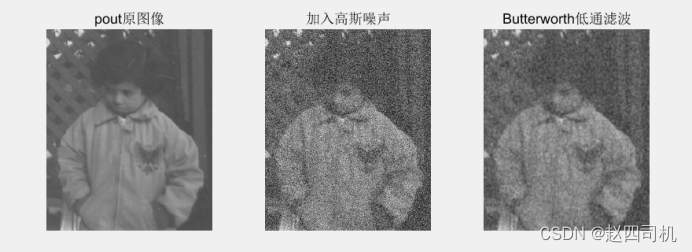``````X2_2=Butterworth_low(I2_2);

figure,subplot(131),imshow(I2),title('pout原图像');

subplot(132),imshow(I2_2),title('加入椒盐噪声');

subplot(133),imshow(X2_2),title('Butterworth低通滤波');``````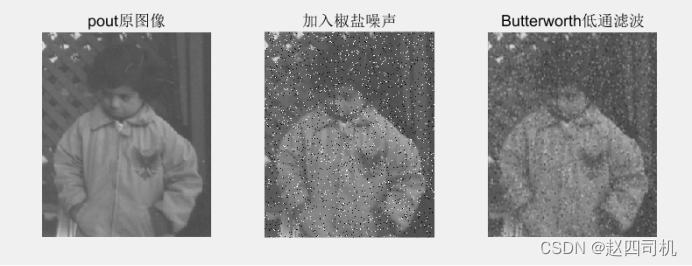``````X2_3=Butterworth_low(I2_3);

figure,subplot(131),imshow(I2),title('pout原图像');

subplot(132),imshow(I2_3),title('加入泊松噪声');

subplot(133),imshow(X2_3),title('Butterworth低通滤波');``````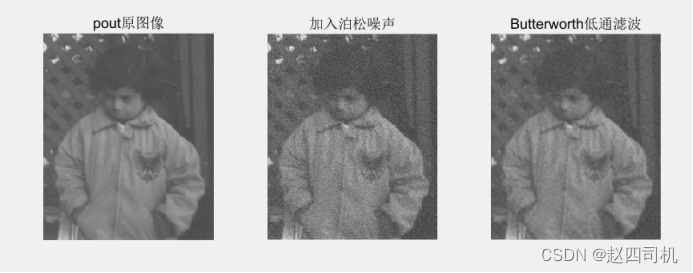``````%自定义scenery图像

X3_1=Butterworth_low(I3_1);

figure,subplot(131),imshow(I3),title('scenery原图像');

subplot(132),imshow(I3_1),title('加入高斯噪声');

subplot(133),imshow(X3_1),title('Butterworth低通滤波');``````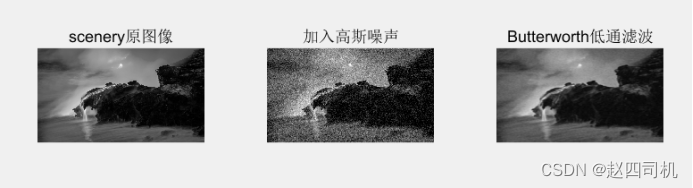``````X3_2=Butterworth_low(I3_2);

figure,subplot(131),imshow(I3),title('scenery原图像');

subplot(132),imshow(I3_2),title('加入椒盐噪声');

subplot(133),imshow(X3_2),title('Butterworth低通滤波');``````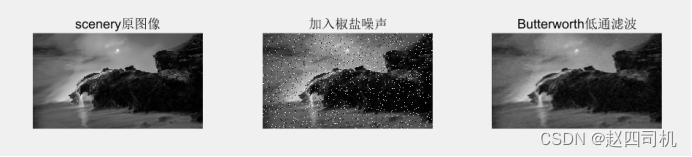``````X3_3=Butterworth_low(I3_3);

figure,subplot(131),imshow(I3),title('scenery原图像');

subplot(132),imshow(I3_3),title('加入泊松噪声');

subplot(133),imshow(X3_3),title('Butterworth低通滤波');``````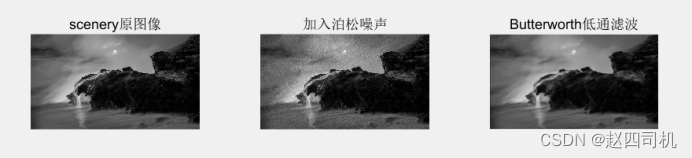## 3.Butterworth高通滤波器

``````function I = Butterworth_high(I_in)

f=double(I_in);

g=fft2(f);     %采用傅立叶变换

g=fftshift(g); %数据矩阵平移

[N1,N2]=size(g);

result=zeros(N1,N2);

n=2;

d1=5;

n1=fix(N1/2);

n2=fix(N2/2);

for i=1:N1

for j=1:N2

d=sqrt((i-n1)^2+(j-n2)^2);

%计算Butterworth低通变换函数

if d==0

h=0;

else

h=1/(1+(d1/d)^(2*n));

end

result(i,j)=h*g(i,j);

end

end

result=ifftshift(result);

X2=ifft2(result);

I=uint8(real(X2));

end``````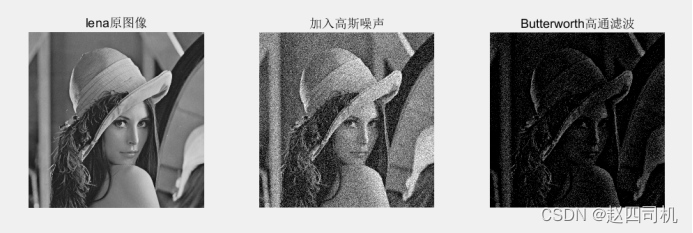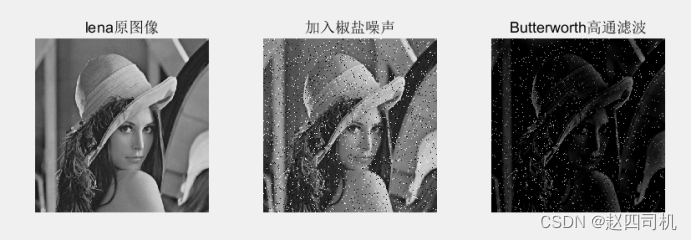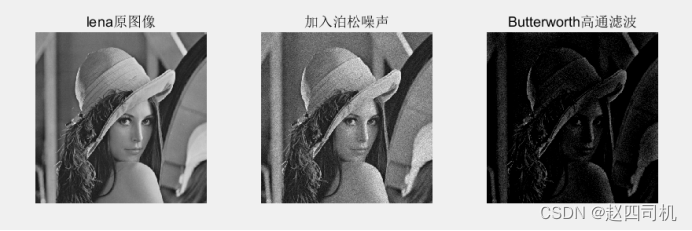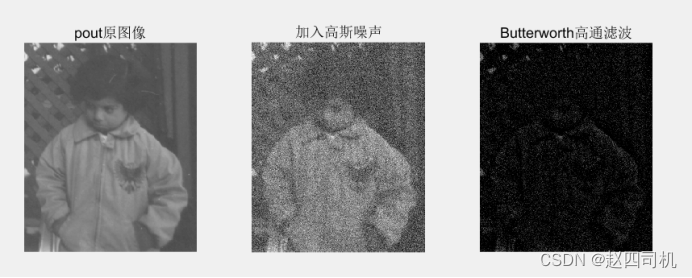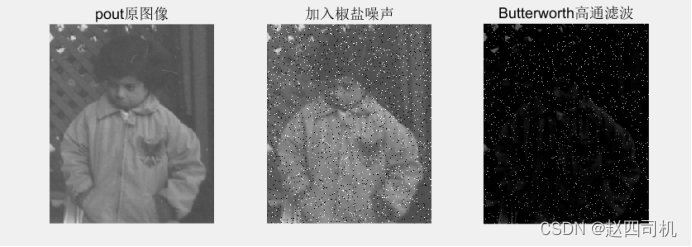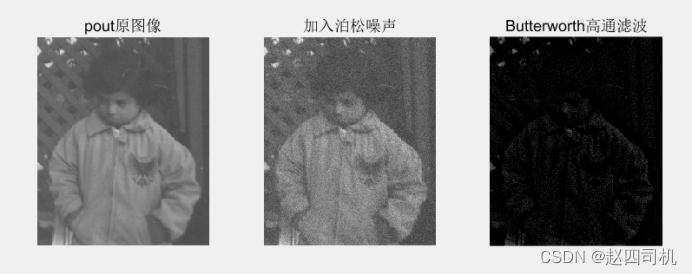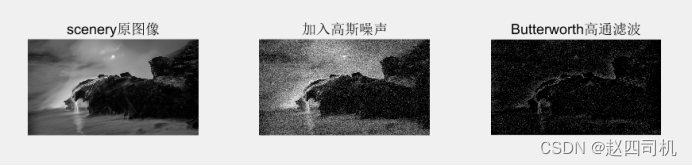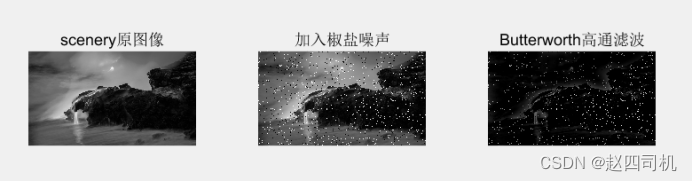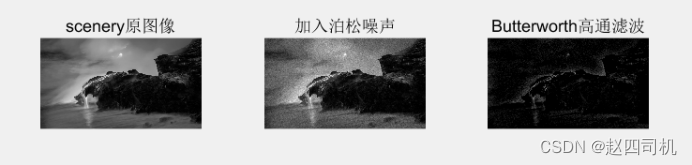## 4.实验分析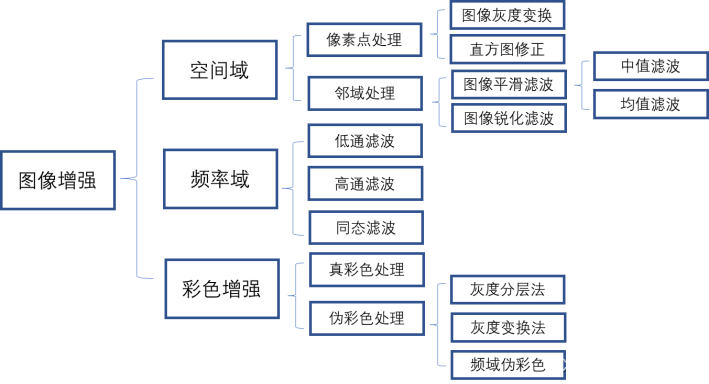一开始我的理解为对于二值图像，当某一像素点与中心点的距离大于D0时，该点的像素值就会被置0，因为根据理想低通滤波函数当D>D0时H=0，当D<=D0时，H=1，而G(u,v)=H(u,v)F(u,v)，由于这时候做的是频域上的处理，故不需要进行卷积操作，只需要做乘积操作。这就是问题的关键所在，乘积操作是作用在频域上的，频域上的点不一一和空域上的点对应，所以滤波后的结果并不是说距离中心点距离大于D0就置0。而对应于空域，可将h(x,y)分为两部分：原点处的中心部分，中心周围集中的成周期分布的外围部分。前者决定模糊，后者决定振铃现象。理想低通滤波器函数转换为空域后，对应的函数图像两边对称的余波就是造成振铃现象的原因。

低通滤波器，顾名思义就是将高频的图像信息过滤掉让低频部分通过，由于低频部分表示图像变化缓慢的部分，故低通滤波器可以实现过滤噪声，平滑图像的功能。低通滤波器有多种，理想的低通滤波器非常尖锐，其在以原点为圆心、D0为半径的园内，通过所有的频率，而在圆外截断所有的频率。从上面实验结果可以看到，布特沃斯低通滤波对于各种噪声的去除效果还是比较理想的，但是实验中发现对变化较平缓的图像（如pout图像），无论是加入高斯噪声还是椒盐噪声，布特沃斯低通滤波的去噪效果并不明显（见图2.1-4）。我猜想其中的原因是由于图像加入噪声之后绝大部分也还是低频信息，通过布特沃斯低通滤波器后绝大多数的噪声未能被过滤掉。因此可以考虑将截止频率D0调低，将D0由50调至30，可以有效去除噪声，但是随之而来的是图像的模糊问题（见下图）。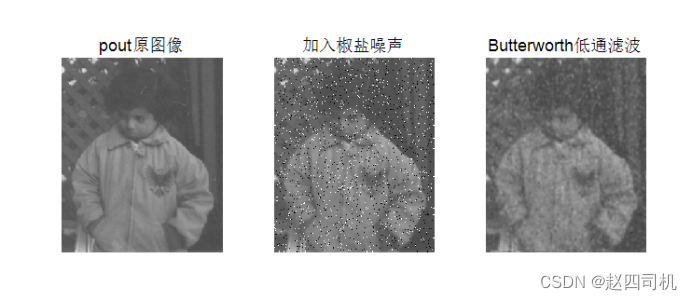除此之外，当布特沃斯低通滤波器的阶很大时（截止频率也要较小），这是其效果就接近理想低通滤波器，会产生振铃现象（见下图）。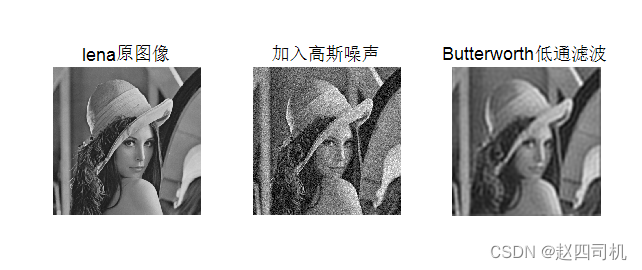对于布特沃斯高通滤波，其作用是过滤掉图像的低频部分，保留图像的高频部分。由于图像的高频部分对应着图像的边缘，所以通过高通滤波后的效果是得到图像的边缘，达到图像锐化的作用。由于图像噪声对应着高频部分，所以高通滤波会把噪声保留下来。对于变化平缓的图像（如pout图像），在参数不变的情况下可以看到加入椒盐噪声后锐化效果不明显（得到的边缘不清晰，见图2.2-5）。这是就需要调整参数，以改变其对边缘检测的“灵敏度”，这就说明要降低截止频率，让更多的相对低频的信息能通过，将截止频率由5降至3，得到结果见下图，可以看到图像的边缘更清晰了一些，尽管则会导致噪声的增加。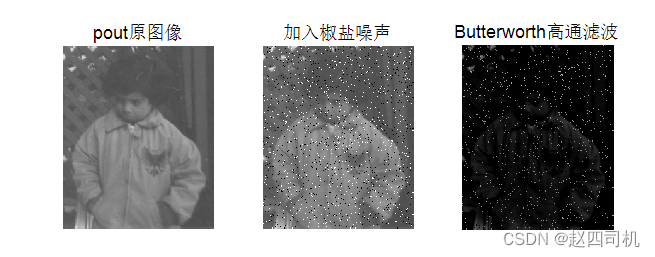均值滤波、中值滤波等都是直接在空域上对图像进行处理，而布特沃斯低通滤波器是在频域上对图像进行处理，所以在处理上面布特沃斯低通滤波器的处理过程要更“细腻”一些。对于不同的噪声，布特沃斯都能带来较好的降噪效果，而对于空域上面的平滑滤波，均值滤波会导致图像的边缘模糊化，中值滤波可能会改变图像的性质。

(0)